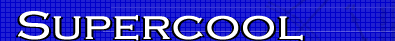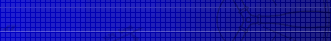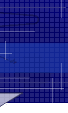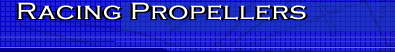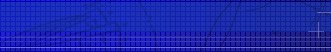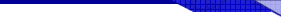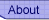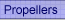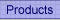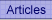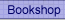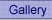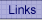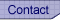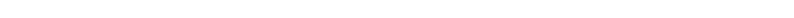﻿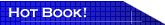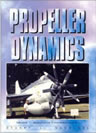Propeller Dynamics Essential reading for model aircraft contest fliers. This is the only book on the market explaining propeller theory in non-mathematical terms. A rattling good read, I know, I wrote it. Read more...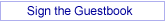## C/L: Speed model C/G position

By Joe Supercool

The position of the centre of gravity (C/G, balance point) on control line speed models has always been a mystery to me. As a F/F guy in the main, to find the C/G close up by the leading edge is a real puzzle. The same applies in spades to monoline; also to F2C team race models.

In analysing aircraft, it is common to treat the machine as being supported by lift at the aerodynamic centre, which is usually at the 25% chord position, where also operates a pitching moment caused primarily by the wing camber. This latter pitching moment is usually balanced by an opposing moment generated by the tailplane. The drag associated with the participation of the tailplane is called "trim" drag, which is undesirable. Placing the balance point at the 25% position can minimise the trim drag.

But with control-line speed models, we have a strange business indeed. The airfoil section is commonly close to symmetrical, which means low camber and low pitching moment. So you would think that the C/G would logically be placed near the 25% chord position, with the tailplane loafing along behind.

But it is not. Indeed, the C/G can be within 5% of the leading edge. This apparent nose-heavy condition should require lots of up-elevator in flight, with lots of trim drag as a result. But that does not happen. My observation is that at take-off and landing, lots
of up-elevator is needed, but not in level flight. This is all very weird, a puzzle for those driving 5000 km from Perth to the 63rd MAAA Albury-Wodonga Nats in 2009.

Indeed, on the dead straight 90 mile section, one does not even need to turn the steering wheel. So with the A/C directed at my face and with Vera Lynn on the C/D player, I ignored the 40C plus heat outside on the Nullabor and gave my brain free rein on this problem.

This is my analysis. The rotating masses of the propeller and crankshaft have angular momentum, thereby producing the behaviour of a gyroscope. Gyroscopes are wonderful things, with weird behaviour. So you see, we are on the right track thinking this way. The weirdness is this. If you push on the gyroscope's axis of rotation, the wretched thing does not move the way you want. Indeed, the axis wants, perversely, to move at right angles to the push. This is strange indeed.

Here is the rule of gyroscopes. The reaction to a push on the axis of rotation takes place 90 degrees later in the direction of rotation. The resultant motion is called precession. Now back to our speed model.

The axis of rotation of our propeller is changing direction all the time. I mean, it is going around in a circle, and pretty quickly at that! The result is a nose up pitching moment. Thats right. Just because the model is flying in a circle and has a gyroscope up
front, the nose wants to lift. You would need to give down elevator to stop this, but that then introduces trim drag, which we don't want.

So by making the model nose-heavy, with the C/G well forward, we can counter the pitch-up without using the elevator. Now that is really neat.

But is it true? As it happens, we can put some numbers into this hypothesis to see if we are on the right track. There is a simple formula for the moment M of a gyroscope. With I the moment of inertia, w1 the angular velocity of the gyro and w2 the angular velocity of the gyro axis, we have:

M = I . w1 . w2

Hmm, what is all that about. Well I is to rotation as mass is to linear motion. That is, moment of inertia is like mass, but the object is spinning. As an approximation, for a propeller of mass m and length L we can pretend the propeller is a slender rod. Then the
propeller has moment of inertia:

I = m . L . L / 12

For a rough calculation for an F2A setup, we can take the mass of the rod (propeller) to be .002 Kg ( 2 grams), and the length 0.15 m (150 mm). Then, ignoring the weight of the crankshaft:

I = 0.002 x 0.15 x 0.15 / 12
= 0.00000375 Kg.m.m

Now F2A engines turn about 40000 RPM, so:

w1 = 2 * pi * RPM / 60

Like wise, the model sweeps thru 2 * pi radians every 1.4 seconds (roughly 290 kph), so that:

w2 = 2 * pi / 1.4

(2 * pi radians is 360 degrees)

Finally, the gyroscopic turning moment is:

M = 0.00000375 * 4200 * 4.49
= 0.071 Kg/m

So far so good. We have an estimate of the nose-up tendency resulting from the gyroscopic effect. I have no sense of the size of this number, except that we need a similar sort of number to counteract and hence balance this moment.

The counter-balancing moment comes from having the C/G well forward, say a distance "x" from the aerodynamic centre. Let us call this moment B for want of something better. Then the condition for balance is:

M = B

Whoa, aint maths marvellous!

Well B is a moment, like a lever, so we then have:

B = mg . x

Here mg is the weight force, being the mass of the airplane times the acceleration due to gravity. I don't know what an F2A airplane weighs, but lets guess its mass is 0.42 Kg, and recall that g is 9.8 m/s/s, so that:

mg = 0.42 * 9.8
= 4.12 Newtons (force units)

Going back to M = B and substituting,

4.12 * x = .071
x = 0.017 m
= 17 mm

So there needs to be a nose-heavy condition, placed 17mm forward to, balance the gyro nose-up tendency. Well, I reckon that places the C/G close to the leading edge. Indeed, with a heavy prop and spinner, the C/G could even be in front of the leading edge!

So there we have it. The C/G miles forward, the nose held up by gyroscopic force and tailplane loafing along behind, doing nothing! Fun things, these speed models.

Now I ask you. Is that not cool?